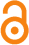Generalized Einstein’s Theory, Gravitodynamics, and Generalized Newton’s Theory of Negative Gravitational Mass/Gravitational Charge

ORCID 0000-0002-1844-3163

Abstract

The purpose of this article is to identify laws that would describe gravitation if negative gravitational mass/gravitational charge (g-charge) exists. WEP is generalized to establish correlations between mass and positive/negative g-charge (Q_(g+)/Q_(g-)), although Q_(g-) on Earth would fall upward. Newton’s theory and Einstein’s theory are generalized to describe behaviors of objects carrying Q_(g-). A significant conclusion is that, as long as Q_(g-) is introduced, all of Newton’s theory, Gravitodynamics and Einstein’s theory explain the accelerated expansion of Universe equally well without needs of “negative pressure” and “cosmological constant” that has fine-tuning issue. This implies that this mechanism of the cosmological phenomenon is universal and theory independent, which strongly supports the existence of negative g-charges. Moreover the acceleration is increasing with time. Gravitodynamics and generalized Einstein’s theory predict: (1) gravitational wave (GW_-) emitted by Q_(g-); (2) GW_- cannot be distinguished from GW_+ emitted by Q_(g+).

Keywords: repulsive gravitation, accelerated expansion of Universe, Newton’s theory of gravity, Gravitodynamics, Einstein’s theory of gravity.

Citation: Peng, H. (2016). Generalized Einstein’s Theory, Gravitodynamics, and Generalized Newton’s Theory of Negative Gravitational Mass/Gravitational Charge. Open Science Repository Physics, Online(open-access), e45011852. http://doi.org/10.7392/OPENACCESS.45011852

Published: September 19, 2016

Contact: research@open-science-repository.com

Full text

Cite this paper

APA

Peng, H. (2016). Generalized Einstein’s Theory, Gravitodynamics, and Generalized Newton’s Theory of Negative Gravitational Mass/Gravitational Charge. Open Science Repository Physics, Online(open-access), e45011852. http://doi.org/10.7392/OPENACCESS.45011852

MLA

Peng, Hui. “Generalized Einstein’s Theory, Gravitodynamics, and Generalized Newton’s Theory of Negative Gravitational Mass/Gravitational Charge.” Open Science Repository Physics Online.open-access (2016): e45011852. Web.

Chicago

Peng, Hui. “Generalized Einstein’s Theory, Gravitodynamics, and Generalized Newton’s Theory of Negative Gravitational Mass/Gravitational Charge.” Open Science Repository Physics Online, no. open-access (2016): e45011852. doi:10.7392/OPENACCESS.45011852.

Harvard

Peng, H. (2016) ‘Generalized Einstein’s Theory, Gravitodynamics, and Generalized Newton’s Theory of Negative Gravitational Mass/Gravitational Charge’, Open Science Repository Physics. Open Science Repository, Online(open-access), p. e45011852. doi: 10.7392/OPENACCESS.45011852.

Science

1. H. Peng, Generalized Einstein’s Theory, Gravitodynamics, and Generalized Newton’s Theory of Negative Gravitational Mass/Gravitational Charge, Open Sci. Repos. Phys. Online, e45011852 (2016).

Nature

1. Peng, H. Generalized Einstein’s Theory, Gravitodynamics, and Generalized Newton’s Theory of Negative Gravitational Mass/Gravitational Charge. Open Sci. Repos. Phys. Online, e45011852 (2016).

doi

Research registered in the DOI resolution system as: 10.7392/openaccess.45011852.